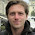## Sunday, July 8, 2012

### Voronoi cells

Recently, I have implemented the diamond-cutting algorithm to calculate the Voronoi cell of a lattice as described in the paper Computing the Voronoi Cell of a Lattice: The Diamond-Cutting Algorithm by Emanuele Viterbo and Ezio Biglieri.

Basically, it starts with a parallelotope defined by hyperplanes through (and normal to) the halves of the basis vectors (and their negated counterparts). Then it gradually combines basis vectors and cuts off  further half spaces, much like a diamond is cut.

The algorithm considers vectors within a certain distance from the origin. This radius can be specified explicitly to speed things up significantly; if not, a "conservative" safe choice is made.

In the current implementation, I am using Sage's existing Polyhedron class to perform the cutting. It seems reasonably fast.

Emanuele Viterbo was so friendly as to provide his implementation of the algorithm from 1995. Comparing running times, it does not seem like it is worth switching from the Polyhedron class to a custom-tailored version of cutting (i.e. intersecting polyhedrons with half spaces). For example, calculating the Voronoi cell of the following lattice:
```2.45 0.00 0.00 0.00 0.00 0.00
-0.41 2.42 0.00 0.00 0.00 0.00
-0.41 -0.48 2.37 0.00 0.00 0.00
-0.41 -0.48 -0.59 2.29 0.00 0.00
-0.41 -0.48 -0.59 -0.76 2.16 0.00
-0.41 -0.48 -0.59 -0.76 -1.08 1.87
```
with ball radius 3 takes 51.4 sec using Emanuele's program but only 13.5 sec with my implementation using Polyhedron on my 2.4 GHz Intel Core 2 Duo Mac. (104 cutting operations are performed in both cases.)

A nice consequence of using the Polyhedron class is that it already provides methods to deal with the resulting Voronoi cell. For example, a representation either by (in)equalities or by vertices/edges/faces can be computed easily.

I had to take special care to deal with non-quadratic basis matrices. To deal with "over-specified" lattices (e.g. redundant basis vectors as in (1,0), (2,0), (0,1)), an LLL-reduction is performed prior to diamond-cutting. (This helps to speed up the algorithm anyway.)

"Non-full" lattices (less basis vectors than dimension of embedded space) are dealt with in a special way, too:
1. To get a quadratic basis matrix, "artificial" basis vectors that represent infinity are added first (they are simply chosen to be longer than any other basis vector);
2. LLL-reduction is performed to get a quadratic basis matrix.
3. After diamond-cutting, all inequalities induced by artificial basis vectors are removed again.
This allows the following:

```sage: L = Lattice([[2, 0, 0], [0, 2, 0]])
sage: V = L.voronoi_cell()
sage: V.Hrepresentation()
(An inequality (-1, 0, 0) x + 1 >= 0, An inequality (0, -1, 0) x + 1 >= 0, An inequality (1, 0, 0) x + 1 >= 0, An inequality (0, 1, 0) x + 1 >= 0)```

Of course, this is still sort of a workaround—maybe there is a better solution to the problem of non-quadratic basis matrices.

1.Is this diamond cutting algorithm implemented on sage 5.1?

1.No, sorry, this is not in Sage 5.1. The whole project dealing with lattices is still ongoing. It will be finished by the end of August. Then it will be reviewed and integrated into Sage as a whole. In the meanwhile, you can check out my code at the github repository: https://github.com/poeschko/sage

2.Hi Jan,
Is your code applicable for disordered lattices? For example, a planar graph?

Best
Sumantra

3.Hey! Were you able to fulfill all the settings of this site on your own or you turned to professionals to receive help?

4.Hi Jan,

Could please tell me that your project on lattices is integrated into Sage or not? I am trying to run your code, but getting many errors. For example the line

from sage.geometry.polyhedron.constructor import Polyhedron

gives an error because there is no file named constructor in the folder geometry.polyhedron. it only contains one file named as base.py

Regards

5."Non-full" lattices (less basis vectors than dimension of embedded space) are dealt with in a special way ...."

These is a faster solution to this:

Let M be m x n lattice generator matrix with n>m
(rows are basis vectors).
1) compute G = M*M^t
2) computing the Cholesky decomposition of G=L*L^t (L lower triangular), yields a square lattice generator matrix L

6.nice post thanks
appvn